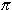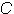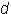# Diameter, Radius, and Circumference of a Circle

The distance around a circle is called the circumference. The distance across a circle through the center is called the diameteris the ratio of the circumference of a circle to the diameter. Thus, for any circle, if you divide the circumference by the diameter, you get a value close to. This relationship is expressed in the following formula:whereis circumference andis diameter. You can test this formula at home with a round dinner plate. If you measure the circumference and the diameter of the plate and then divideby, your quotient should come close to. Another way to write this formula is:where · means multiply. This second formula for finding the circumference of a circle is commonly used in problems where the diameter is given and the circumference is not known (see the examples below).

For more help, check out our Circumference of a Circle Lesson Plan